Function Repository Resource:

# LogContract

Contracts sums of logarithms into a single logarithm

Contributed by: Carl Woll
 ResourceFunction["LogContract"][expr] contracts sums of Log expressions into a single Log expression.

## Details and Options

ResourceFunction["LogContract"] converts aLog[b] to Log[ba] plus an extra term that depends on the branch cut of Log for the given arguments.
ResourceFunction["LogContract"] converts Log[a]+Log[b] to Log[ab] plus an extra term that depends on the branch cut of Log for the given arguments.
ResourceFunction["LogContract"] automatically threads over lists, as well as equations, inequalities and logic functions.
Products that contain a Log expression are converted into an equivalent Log expression where the factor is included as the exponent.
ResourceFunction["LogContract"] has the option Assumptions, specifying assumptions to use.
The default setting for the Assumptions option is True, corresponding to no assumptions.
With the setting Automatic for the Assumptions option, a maximal set of assumptions is made, disregarding all issues of branches of multivalued functions.

## Examples

### Basic Examples (3)

Reproduce a basic Log identity:

 In:=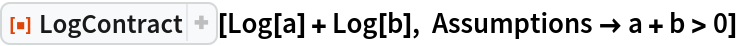Out=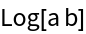Find conditions for which Log[a]-Log[b] is equal to Log[a/b]:

 In:=Out=Contract logs, disregarding branch cut issues:

 In:=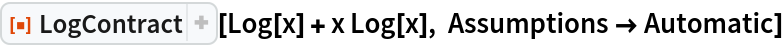Out=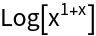The result is not generically true:

 In:=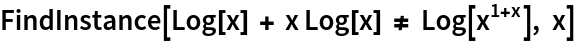Out=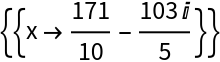### Scope (2)

 In:=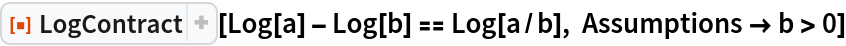Out=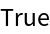LogContract is essentially the inverse of PowerExpand for logarithmic expressions:

 In:=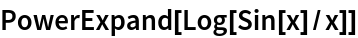Out=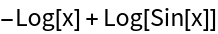Contracting to the original expression:

 In:=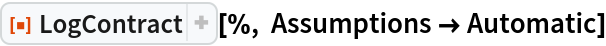Out=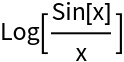### Options (2)

#### Assumptions (2)

The default option setting for Assumptions is \$Assumptions. By default , meaning that LogContract gives a universally correct result:

 In:=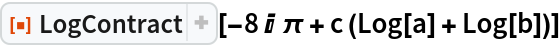Out=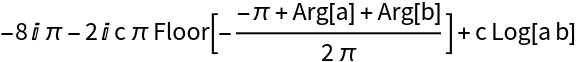With the setting Automatic, a maximal set of assumptions are used and the output is not generically correct:

 In:=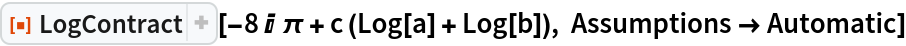Out=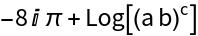A simpler result is obtained with suitable assumptions:

 In:=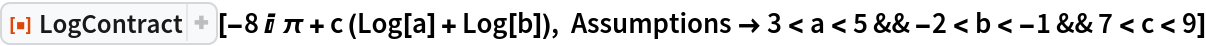Out=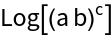Contract logs assuming real arguments:

 In:=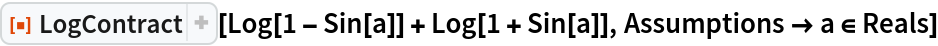Out=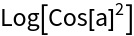In:=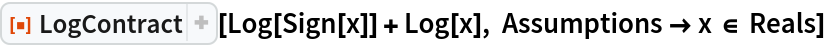Out=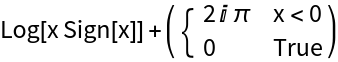In:=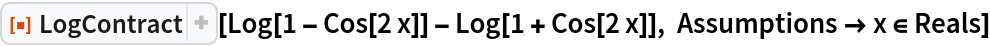Out=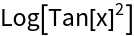### Possible Issues (1)

The default option for LogContract is , unlike PowerExpand which uses Automatic as the default:

 In:=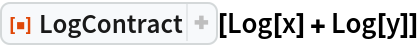Out=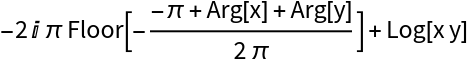In:=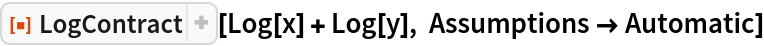Out=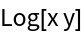Carl Woll

## Version History

• 1.0.0 – 22 October 2019